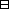## Group Theory and Linear Algebra

Last updated: 23 September 2014

## Lecture 17: The Spectral Theorem

Let $V$ be a finite dimensional vector space. Let $⟨,⟩:V×V\to ℂ$ be a positive definite Hermitian form. Let $f:V\to V$ be a linear transformation. The adjoint to $f$ is ${f}^{*}:V\to V$ such that if $u,w\in V$ then $⟨f\left(u\right),w⟩=⟨u,{f}^{*}\left(w\right)⟩\text{.}$

The linear transformation $f$ is

 $•$ self adjoint, or Hermitian, if $f$ satisfies $f=f*,$ $•$ an isometry, or unitary, if $f$ satisfies $f*f=1,$ $•$ normal, if $f$ satisfies $f*f=ff*.$

Let $A$ be an $n×n$ matrix. The matrix $A$ is

 $•$ self adjoint, or Hermitian, if $A$ satisfies $A=A‾t,$ $•$ an isometry, or unitary, if $A$ satisfies ${\stackrel{‾}{A}}^{t}A=1,$ $•$ normal, if $A$ satisfies $AA‾t= A‾tA.$

Let $V$ be an inner product space and let $B=\left\{{b}_{1},{b}_{2},\dots ,{b}_{k}\right\}$ be an orthonormal basis of $V\text{.}$ Then $C= { f(b1), f(b2),…, f(bk) }$ is an orthonormal basis of $V$ if and only if $f$ is unitary.

(Spectral Theorem). Let $V$ be an inner product space and let $f:V\to V$ be a normal linear transformation. Let $B=\left\{{b}_{1},\dots {b}_{k}\right\}$ be an orthonormal basis of $V$ and let $A={B}_{f}$ be the matrix of $f$ with respect to $B\text{.}$ Then, there exists a unitary matrix $P$ such that $PAP-1$ is diagonal.Idea of proof. Show that $A$ and ${\stackrel{‾}{A}}^{t}$ have a common eigenvector ${v}_{1}$ (so that $A{v}_{1}=\lambda {v}_{1}$ and ${\stackrel{‾}{A}}^{t}{v}_{1}=\mu {v}_{1}\text{).}$ Let ${U}_{1}=\text{span}\left\{{v}_{1}\right\}$ and write $V={U}_{1}\oplus {U}_{1}^{\perp }\text{.}$ Show that $A$ and ${\stackrel{‾}{A}}^{t}$ have a common eigenvector ${v}_{2}\in {U}_{1}^{\perp }\text{.}$ Let ${U}_{2}=\text{span}\left\{{v}_{2}\right\}$ and write $V={U}_{1}\oplus \left({U}_{2}\oplus {U}_{2}^{\perp }\right)\text{.}$ Continue to get $C=\left\{{v}_{1},{v}_{2},\dots ,{v}_{k}\right\}\text{.}$ $\square$

Let $A= ( 001 100 010 ) ,ζ=-1+3i2, ζ2=-1-3i2.$ Then $A‾t= ( 010 001 100 ) and AA‾t= ( 100 010 001 ) =A‾tA.$ So $A$ is a normal matrix. Then ${v}_{1}=\left(\begin{array}{c}1\\ 1\\ 1\end{array}\right)$ is an eigenvector $A{v}_{1}={v}_{1}\text{.}$ If ${U}_{1}=\text{span}\left\{\left(\begin{array}{c}1\\ 1\\ 1\end{array}\right)\right\}$ then $U1⊥= { (a1a2a3) | a1+a2+a3=0 } .$ Then ${v}_{2}=\left(\begin{array}{c}1\\ \frac{-1-\sqrt{3}i}{2}\\ \frac{-1+\sqrt{3}i}{2}\end{array}\right)$ is an eigenvector $A{v}_{2}=\zeta {v}_{2}\text{.}$ $U2=span{v2}=span {(1-1-3i2-1+3i2)}$ is a subspace of ${U}_{1}^{\perp }$ and its complement in ${U}_{1}^{\perp }$ is $U2⊥ = { (a1a2a3) | a1+a2+a3=0, a1‾+ζ2a2‾+ζa3‾=0 } = span{(1ζζ2)}$ since $\text{dim}\left({U}_{2}^{\perp }\right)=1$ and $1+{\zeta }^{2}·{\zeta }^{2}+\zeta ·\zeta =1=\zeta +{\zeta }^{2}=0\text{.}$ (Note: $\stackrel{‾}{\zeta }={\zeta }^{2}$ and $\stackrel{‾}{{\zeta }^{2}}=\zeta \text{).}$ Let ${v}_{3}=\left(\begin{array}{c}1\\ \zeta \\ {\zeta }^{2}\end{array}\right)$ with respect to the basis $\left\{{v}_{1},{v}_{2},{v}_{3}\right\}=B,$ $Ba= ( 100 0ζ0 00ζ2 ) .$ If $P=13 ( 111 1ζ2ζ 1ζζ2 )$ is the change of basis matrix from $S=\left\{{e}_{1},{e}_{2},{e}_{3}\right\}$ with ${e}_{1}=\left(\begin{array}{c}1\\ 0& 0\end{array}\right),$ ${e}_{2}=\left(\begin{array}{c}0\\ 1& 0\end{array}\right),$ ${e}_{3}=\left(\begin{array}{c}0\\ 0& 1\end{array}\right)$ to $B=\left\{{v}_{1}^{\prime },{v}_{2}^{\prime },{v}_{3}^{\prime }\right\}$ with ${v}_{1}^{\prime }=\frac{1}{\sqrt{3}}\left(\begin{array}{c}1\\ 1\\ 1\end{array}\right),$ ${v}_{2}^{\prime }=\frac{1}{\sqrt{3}}\left(\begin{array}{c}1\\ {\zeta }^{2}\\ \zeta \end{array}\right),$ ${v}_{3}^{\prime }=\frac{1}{\sqrt{3}}\left(\begin{array}{c}1\\ \zeta \\ {\zeta }^{2}\end{array}\right)$ so that both $S$ and $B$ are orthonormal, then $P-1=P‾t=13 ( 111 1ζζ2 1ζ2ζ ) ,$ since $P$ is unitary, and $PAP-1= ( 100 0ζ0 00ζ2 )$ is diagonal.

## Notes and References

These are a typed copy of Lecture 17 from a series of handwritten lecture notes for the class Group Theory and Linear Algebra given on August 31, 2011.